## Thursday, 19 February 2015

### Unity3D Shaders–Surface Shaders : Lighting Models

Continuing with surface shaders, in this post I am going to look at how you can create your own lighting models.
Now, lighting models are just the ability to calculate how the light(s) bounces off the surface of the geometry. It does not the ability to do your own shadow mapping.
So, as I said in an earlier post, we declare the lighting model to be used in the shader, in the #pragma section, in all our other surface shaders we have used the Lambert lighting model, we can also use BlinnPhong.
`  #pragma surface surf Lambert`

Lighting models can be written for both forward and deferred rendering pipelines, you can alter the render mode in Unity, by default it uses forward rendering and I think deferred is a pro feature.

Forward rendering is when each light is calculated against each mesh, so the more lights you have the slower things are going to get. Normally a forward render will not have more than 3 lights in it as after that light calculations start to lag.

Deferred rendering is done once ALL the mesh’s are rendered and they are applied to the whole scene in one go, so with deferred you can have lots and lots of lights will very little slow down.

As Unity uses forward render and deferred it a pro feature I am going to focus on forward surface shader lighting models.

Lets create our own lighting model. To do this we need to give it a name. For this first lighting model we will go with a simple diffuse, so all we want to do in the lighting model is find the angle of the light source bouncing off the surface. As it is a surface shader this done on each pixel of each triangle on the mesh being rendered.

Add a shader to your Shaders asset folder and name is SSDiffuse, create a new material called SSDiffuseMaterial, associate the shdaer with the material as we have done in the previous posts.

It should looks something like this

```Shader "Randomchaos/Tutorial/SSDiffuse"
{
Properties
{
_MainTex ("Base (RGB)", 2D) = "white" {}
}

SubShader
{
Tags { "RenderType"="Opaque" }
LOD 200

CGPROGRAM
#pragma surface surf Lambert

sampler2D _MainTex;
float _Ramp  =1;

struct Input
{
float2 uv_MainTex;
};

void surf (Input IN, inout SurfaceOutput o)
{
half4 c = tex2D (_MainTex, IN.uv_MainTex);
o.Albedo = c.rgb;
o.Alpha = c.a;
}
ENDCG
}
FallBack "Diffuse"
}```

Put a mesh into your scene and apply this shader to it, I have used Dude from the old MS XNA Demos, and with the above shader, he looks like this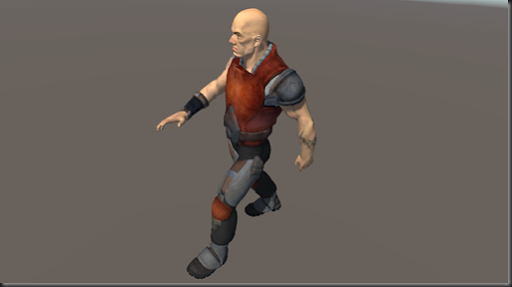Lets set up our own lighting model in this shader. First thing we need to do is tell the shader to use our new lighting model, we well call it SimpleDiffuse and replace Lambert with our new model in the #pragma like this

`  #pragma surface surf SimpleDiffuse`

Now we need to provide a function that gets called to do our lighting calcs, in forward rendering, there are two function signatures we can use, this first is for lighting models that we don’t need to know the cameras view direction, like a diffuse shader, the other is for shaders that do, so things like specular calculations (thanks Unity docs).

`half4 Lighting<Name> (SurfaceOutput s, half3 lightDir, half atten);`

`half4 Lighting<Name> (SurfaceOutput s, half3 lightDir, half3 viewDir, half atten); `

As we can see, the method name needs to start with Lighting followed by the name of our model, in our case SimpleDiffuse, so our function will look like this

```  half4 LightingSimpleDiffuse (SurfaceOutput s, half3 lightDir, half atten)
{
return 1;
}```

If se save our shader now and check out our rendered mesh, it will look like this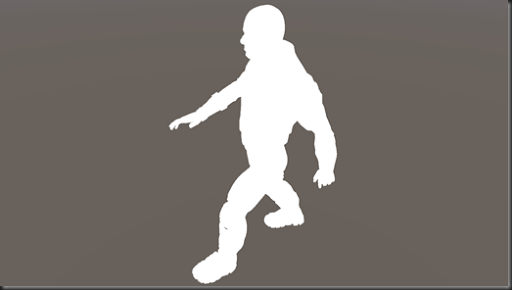The whole thing is white! That’s because, we are returning the value of 1, but the shader is expecting a half4 (half4 is a small float3 effectively), why is there no error thrown?

It’s because when we set a float<n> or half<n> or any other vector to a single value like this, all the elements of the vector are set to that value, so, in this case 1,1,1,1 is returned.

So in our lighting model we are saying that the surface is being lit at every angle with the colour white. If we change our lighting calculation to look like this

```  half4 LightingSimpleDiffuse (SurfaceOutput s, half3 lightDir, half atten)
{
return _LightColor0;
}```

_LightColor0 is pre loaded by Unity for us, this value is set each time the light calculation is done for another light, so this will also handle multiple lights in a forward rendered scene.

We can then play with the lights colour in the scene or game window and we can see that  the texture is still being rendered, but when we set the colour to white in the lighting model, that’s all we see, so the surf shader is being called then the lighting model is being applied to it.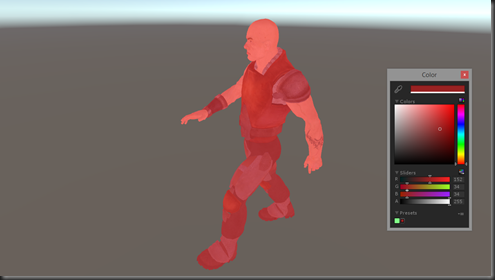OK, lets start to make this shader start to look like a diffuse effect. We can see in our function signature, we are getting passed a structure called SurfaceOutput. This structure gives us a few elements we can use

``` struct SurfaceOutput
{
half3 Albedo;
half3 Normal;
half3 Emission;
half Specular;
half Gloss;
half Alpha;
};```

At the moment in our surface shader we are only setting the Albedoo and the Alpha, so lets use these in conjunction with the light colour like this

```  half4 LightingSimpleDiffuse (SurfaceOutput s, half3 lightDir, half atten)
{
half4 c;

c.rgb = s.Albedo * _LightColor0.rgb;
c.a = s.Alpha;

return c;
}```

So we set up a half4 variable called ‘c’, this is going to be the value we return, we then set the rgb (Red, Green, Blue) elements of the return variable with the given Albedo multiplied by the light colour, why multiplied? Because if we added it and the light was black, we would just get the Albedo color, which would be wrong, if there is no light, no colours should be bouncing off the surface.

We then set the Alpha as it’s passed in, and return what we have calculated.

The effect of this shader now looks like this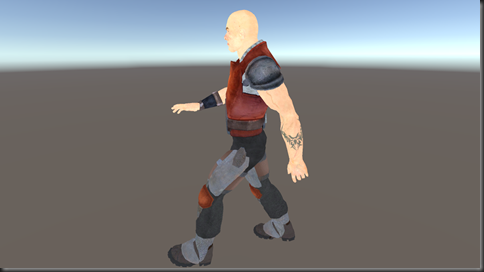So a little better. By the way, if you change your light to black, there is still some light applied to the mesh, this is the ambient light that is applied to the model. We can switch this off, by default, the ambient colour (in Unity 5) is set to that of the skybox, open the Lighting Window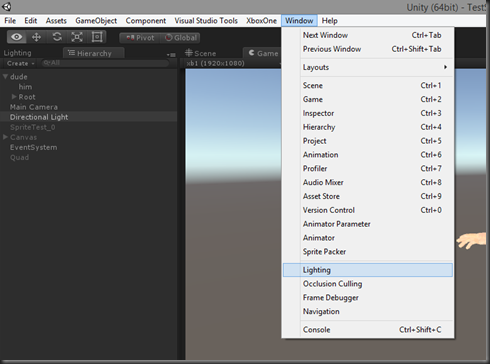You can now either alter the Ambient Source or set it’s intensity to 0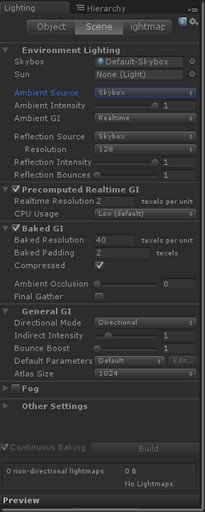Now, our shader is not taking into account the angle of the surface with that of the light, so we get an un natural flat looking render. How do we calculate the angle between the surface and the light, and how do we then modify the colour to reflect (forgive the pun) that?

Well, there is an awesome method we can use called dot. dot(float4, float4) takes two vectors and returns the dot product, of the two vectors. In other words it will return a value between –1 and 1, where 1 would be that the light is directly over the surface, so 90 degrees over it and –1 would effectively be at 90 degrees again but on the other side of the surface, so behind it.

So, how do we do that, how do we know the direction the surface is facing, and the direction of the light hitting that surface?

Well, the light direction we can get easy as Unity hands it to us in our function as a parameter, so that’s that bit solved, but what about the direction the surface is facing….. Remember back in the first post, we covered what a vertex is and the data that is in it?

One of the data elements was called the normal that describes the direction the vertex is facing, so each triangle is made of 3 vertices, each vertex has a normal describing its direction of facing, so, just like with the texture coordinates (described in the second post) these too are interpolated, and if you look at the SurfaceOutput structure, we get given the current pixels “Normal” :) How cool is that!! (I know, I’m a geek)

So, now we can find the angle the light is hitting this pixel of the surface like this

`dot(s.Normal, lightDir)`

Now, this will return a value, as I said above, in the range of –1 and 1, but I don’t care if it’s behind the surface, I just want to know how much light to reflect, so I am going to use a function called saturate that will clamp the value between 0 and 1 and store it in a variable  like this

`   half NdotL = saturate(dot(s.Normal, lightDir));`

We can now use this value to multiply our output colour and our lighting calculation now looks like this

```  half4 LightingSimpleDiffuse (SurfaceOutput s, half3 lightDir, half atten)
{
half4 c;

half NdotL = saturate(dot(s.Normal, lightDir));

c.rgb = s.Albedo * _LightColor0.rgb * NdotL;
c.a = s.Alpha;

return c;
}```

Which then means we get a decent diffuse render like this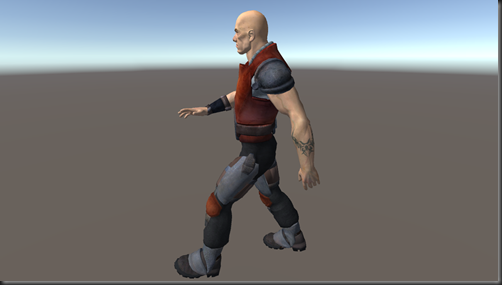So, lets have a look at this with two lights rendered and a floor…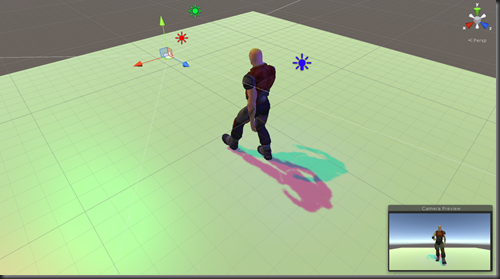As you can see the surface shader is handling all the lighting passes for us, we don’t have to worry about how many lights. In this scene are two  directional lights, one green, one red and a blue point light at the back of the mesh.

I know what you are thinking,

“Why on earth have we just created this shader when I can just use the standard Diffuse shader that ships with Unity?!!!”

Well, I wanted to show you, with the simplest shader I could how we can create our own lighting calculations, and to give you an idea of all the bits Unity is doing for us with a Surface Shader.

I was going to cover more lighting model types and look at some other surface shaders, but I am sure with the small bit of information I have tried to arm you with in these first few posts you can take a look at the examples in the Unity Docs and start experimenting with them your self. You can find the surface shader examples here, and the lighting model examples here.

In my next post, I am going to show how you can combine a surface shader with a vertex shader, using the vertex shader to alter the geometry and also look at a multiple pass surface shader.

As ever, your comments and critique are always welcome, feel free to post :)

<< Previous                                                                                           Next >>